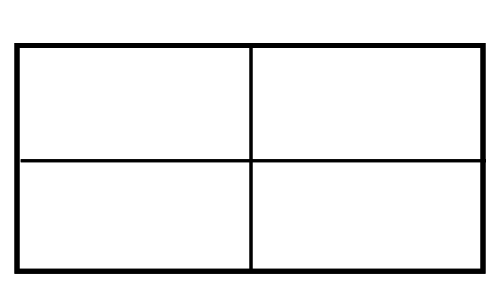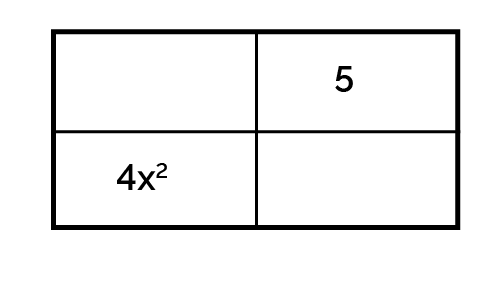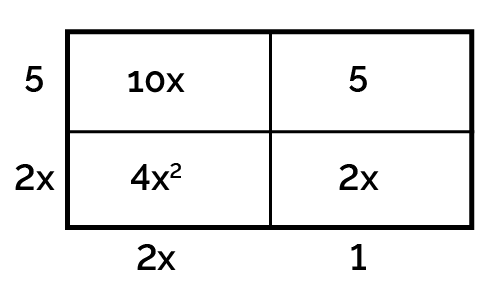# Generic Rectangle Calculator

Reviewed by Anna Szczepanek, PhD and Steven Wooding
Last updated: Jun 05, 2023

If you are new to factoring quadratics using generic rectangles and are looking for a generic rectangle calculator to make your job easier, you have come to the right place. Whether you have a math assignment or are just interested in expanding your knowledge of quadratic equations, we have all the information you need. Keep reading to learn:

• What generic rectangles are;
• What the term factoring means;
• How do generic rectangles help us to solve quadratic equations; and
• How to use our generic rectangle solver.

If you wish to explore other methods used to solve quadratic equations, be sure to check our completing the square calculator, the quadratic formula calculator, and the factoring trinomials calculator.

## What is a quadratic equation?

A quadratic equation is an algebraic equation of a second degree. It is usually written as:

ax2 + bx + c = 0

In this equation, x is an unknown value while a, b, and c are constants. In the quadratic equation, a cannot be equal to 0. The expression at the left-hand side of the equal sign is called a quadratic trinomial.

A quadratic equation can have zero, one, or two solutions (in real numbers). When there is only one solution, it is called a double root.

## What are generic rectangles?

Generic rectangles are rectangles used in mathematics to multiply large numbers. The word generic refers to the fact that we do not draw these rectangles to scale.

We use generic rectangles in this calculator to find the factors of quadratic equations.

🙋 Do you remember what a factor is in mathematics? When we multiply two numbers together to form another number, the first two numbers are called factors of the product.

So, factoring means finding the numbers that can divide a given number without leaving a remainder.

Our generic rectangle solver helps you to find two expressions, which, when multiplied together, will result in the given quadratic trinomial. Then we can immediately answer the question when our trinomial assumes the value zero, which is equivalent to solving a quadratic equation.

## How to factor quadratic equations using a generic rectangle

For our example, we will use the trinomial $4x^2+12x+5 = 0$.

Now before we begin, be sure to draw your generic rectangle like the one shown in the diagram below.If you examine any quadratic trinomial, you will notice that each has a term containing $x^2$ and a constant term (it may be equal to zero!).

Let's begin:

1. First, we place the term containing the $x^2$, in our case $4x^2$, and the constant terms, in again $5$, at opposite ends of the generic rectangle diagonally, like this:1. Next, find the product of $4x^2$ and of the constant term $5$. The answer in this example is $20x^2$.
1. List all the factors of the coefficient of the product found above.

Since this coefficient is $20$ the factors are:

$20$ and $1$
$10$ and $2$
$5$ and $4$

1. Identify the two factors which, when added together, will result in the coefficient of the x term, i.e., in $12$.

As you can see by now, these are $10$ and $2$.

1. Multiply these numbers by $x$ and place them in the empty places in the rectangle:1. Our next task is to find the common factors of each of the smaller sections of our rectangle: of each row and each column. As you can see:

• $5 × 2x = 10x$, so we write $2x$ and $5$ along the length and width of the rectangle containing the $10x$. If you study the diagram below, you will see that the others follow the same pattern.

• $2x × 2x = 4x^2$

• $5 × 1 = 5$ and

• $1 × 2x = 2x$.

We write them outside the rectangle. These are the factors of our equation.1. By looking at what now represents the length and width of the rectangle, we can rewrite our answers as follows:

$(2x + 5)(2x+1)$

Hence, the equation $4x^2 +12x+5 = 0$ takes on the form $(2x + 5)(2x+1) = 0$, which we can solve very easily.

Namely, we must have $2x + 5 = 0$ or $2x+1 = 0$. This means that $x = -\frac 52$ or $x = -\frac 12$. And that is how we solve quadratic equations using generic rectangles!

Do these steps seem too long and complicated for you? Just use our generic rectangle calculator, and you will be done in a jiffy 😁.

## How to use our general rectangle calculator?

Using our generic rectangle solver is quite simple, really. Enter the three constant terms, a, b, c, and our calculator will return the factors and the generic rectangle. It can also provide an explanation of how to factor the equation.

To display a detailed explanation of the steps used to derive the answer, change the default no to yes at the bottom of the calculator.

## FAQ

### What are the factors of the quadratic equation with coefficients 1, 3, and 2 respectively?

(x+2)(x+1).

We factor this equation using generic rectangles. You can verify this answer by expanding the parentheses in the usual manner.

You may also use Omni calculator's generic rectangle calculator to solve the problem quickly.

### How to factor a quadratic equation using a generic rectangle?

To find the factors:

1. Place the term containing x2 at the bottom left of the rectangle and the constant term at the top right corner.

2. Compute their product and list its factors.

3. Find the factors of the x2 term, which, when added together, result in the x term. Put them in empty cells.

4. Find the common factors of each row and each column in the rectangle.

5. The length times the width of the rectangle is the factorization of your equation.

Coefficients
This calculator uses the generic rectangle method to factor the quadratic equation:

ax² + bx + c

with integer coefficients a, b, c.
Enter the coefficients:
a
b
c
Show step-by-step solution?
No, thanks.
People also viewed…

### Completing the square works

Let's discuss why solving quadratic equations by completing the square works!

### Flat vs. round Earth

Omni's not-flat Earth calculator helps you perform three experiments that prove the world is round.

### Meat footprint

Check out the impact meat has on the environment and your health.

### Trapezoid

The trapezoid calculator is here to give you all the information about your trapezoid shape – the sides, height, angles, area, and perimeter.